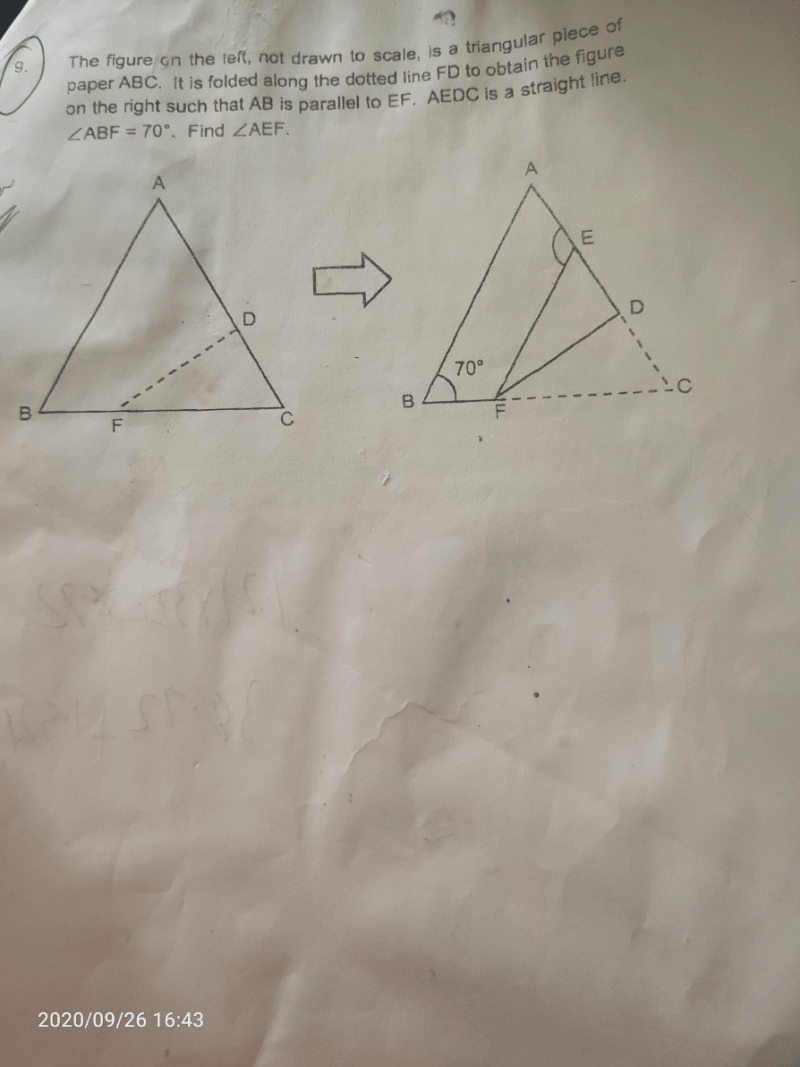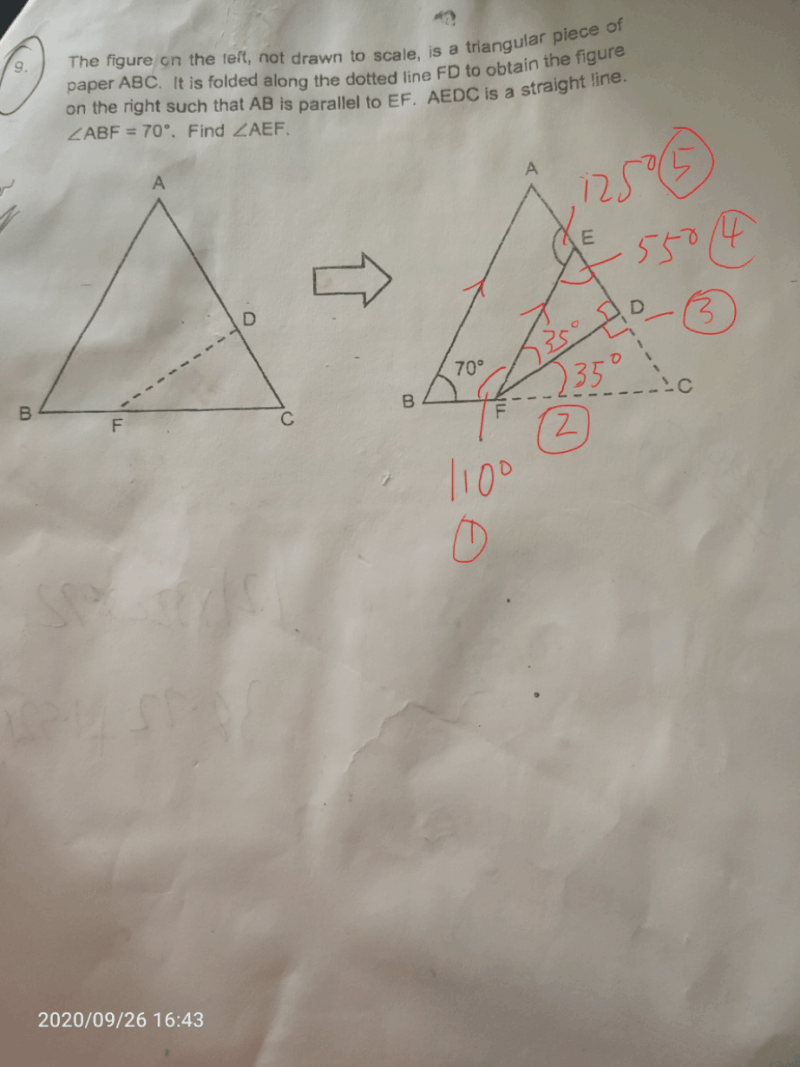# QuestionAEFB is a trapezium with AB parallel to BF.

Angle BFE= 180° – 70° = 110° (interior angles / angles between parallel lines)

Angle EFD = Angle DFC, so Angle EFD = (180° – 70°) ÷ 2 = 35°

Angle EDF = Angle FDC, so Angle EDF = 180° ÷ 2 = 90°

Angle FED = 180° – 35° – 90° = 55°

Angle AEF = 180° – 55° = 125°

Hope you understand and all the best for your PSLE!